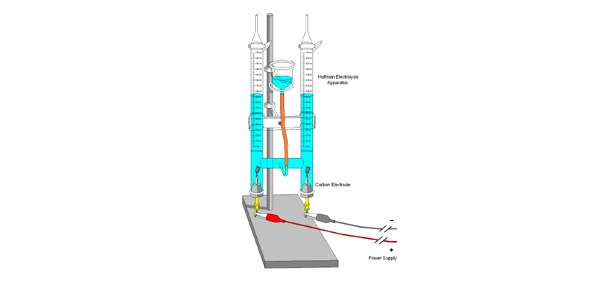# Electrolysis

15 Questions | Total Attempts: 92SettingsThe quiz is basic electrolysis for students who do not know much about electrolysis

Related Topics
• 1.
What is electrolysis
• 2.
Why must the electrodes be inert?
• 3.
The two substances used for electrodes are _________
• 4.
What is the advantage of doing electrolysis in an aqueous solution?
• A.

Low temperature

• B.

More reactive

• 5.
What is the lightest and most abundant element in our universe? ______________
• 6.
What is the most abundant compound on earth? _______________________w
• 7.
What bond is commonly present in this compound?
• A.

Van der Waals

• B.

Hydrogen bonding

• C.

Ionic

• 8.
An anode attracts ______ charged ions.
• 9.
What are the ions present in water?
• 10.
In the electrolysis of molten sodium chloride, what is the yellow green gas formed?
• A.

Chlorine gas

• B.

Bromine gas

• C.

Fluorine gas

• 11.
When water decomposes, is hydrogen gas and oxygen gas formed?
• A.

True

• B.

False

• 12.
During electrolysis, why does some metals want to be in a compound, whereas some wants to be an ion?
• 13.
What is electroplating
• 14.
What is a binary compound?
• 15.
Given below are the electrode equations for the electrolysis of aqueous sodium chloride (brine) and the Ar's: H=1 and Cl=35.5:(-) electrode: 2H+(aq) + 2e- ==> H2(g)(+) electrode: 2Cl-(aq) - 2e- ==> Cl2(g)If 213g of chlorine was formed at the (+) electrode, what volume (dm3) of hydrogen is formed at the (-) electrode? (1 mole of any gas = 24dm3 at room
• A.

72

• B.

144

• C.

36